# Holomorphic mapping

A mapping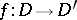of a domain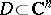into a domain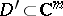under which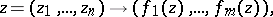where all coordinate functionsare holomorphic in. If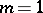, a holomorphic mapping coincides with a holomorphic function (cf. Analytic function).

A holomorphic mapping is called non-degenerate at a point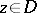if the rank of the Jacobian matrix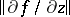is maximal at(and hence equals). A holomorphic mapping is said to be non-degenerate in the domainif it is non-degenerate at all points. If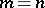, the non-degeneracy ofis equivalent to the condition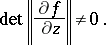If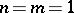, a non-degenerate holomorphic mapping is a conformal mapping. If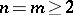, a non-degenerate holomorphic mapping does not, in general, preserve angles between directions. If a holomorphic mappingis non-degenerate at a pointand if, thenis locally invertible, i.e., then there exist neighbourhoods,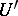,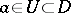,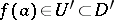, and a holomorphic mapping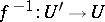such that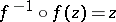for all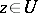. If a holomorphic mappingmapsonto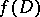in a one-to-one correspondence and if, thenis non-degenerate in; if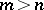, this is not true, e.g.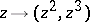,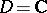,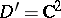. If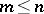and ifis non-degenerate in, then the image ofis also a domain in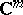; if, the principle of invariance of domain does not hold for mappings that are degenerate at certain points, e.g.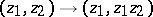,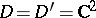.

Ifandare complex manifolds,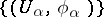and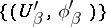are atlases of their local coordinate systems (,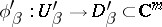are homeomorphisms; cf. Manifold), then a mapping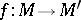is said to be holomorphic if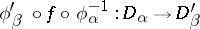is a holomorphic mapping for alland. Holomorphic mappings of complex spaces are defined in a similar manner (cf. Analytic mapping). See also Biholomorphic mapping.